# Transforms

Before reading this, make sure you have an understanding of vectors and how they’re used in game development. If you don’t, I recommend you read this introduction I wrote for the Godot documentation: Vector Math.

## 2D Transforms

In 2D space, we use the familiar X-Y coordinate plane. Remember that in Godot, as in most computer graphics applications, the Y axis points downward: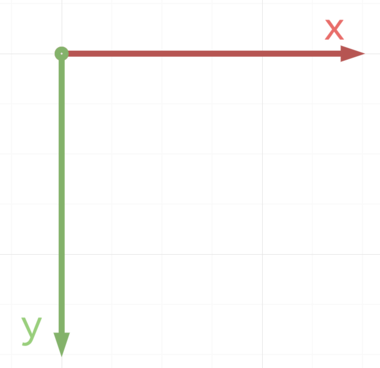To begin, let’s consider this spaceship floating in space: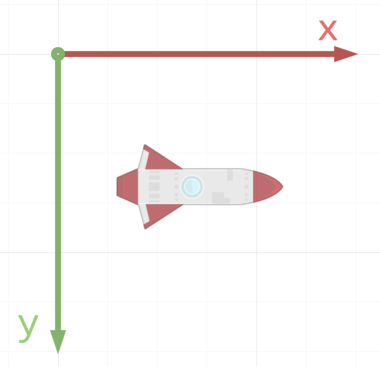The ship is pointing in the same direction as the X axis. If we wanted it to move forward, we could add to its X coordinate and it would move to the right: `position += Vector2(10, 0)`.

But what happens when the ship rotates?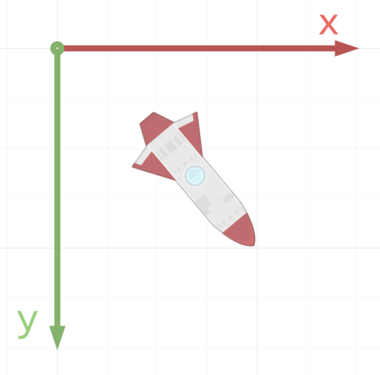How do we move the ship forward now? If you remember Trigonometry from school, you might be starting to think about angles, sin and cos and doing something like `position += Vector2(10 * cos(angle), 10 * sin(angle))`. While this would work, there’s a much more convenient way: the Transform.

Let’s look at the rotated ship again, but this time, let’s also imagine that the ship has its own X and Y axes that it carries with it, independent of the global axes: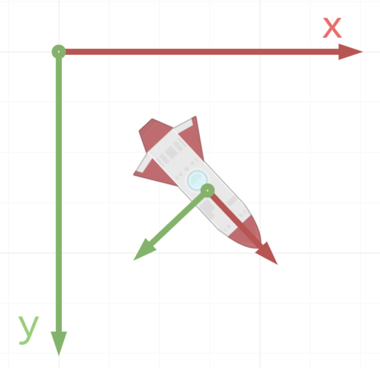These “local” axes are a part of the transform called the `basis`.

Knowing this, we can move the ship forward by moving it along its own X axis and we won’t have to worry about angles and trig functions. To do this in Godot, we can use the `transform` property, which is available to all Node2D derived nodes.

``````    position += transform.x * 10
``````

This code says “Add the transform’s x vector multiplied by 10.” Let’s break down what that means. The `transform` contains `x` and `y` properties that represent those local axes. They are unit vectors, which means their length is `1`. Another term for unit vector is direction vector. They tell us the direction the ship’s x axis is pointing. We then multiply by `10` to scale it to a longer distance.

In addition to the `basis`, the transform also contains a component called the `origin`. The basis represents the body’s rotation, while the origin represents the translation, or change in position.

In this picture, the blue vector is the `transform.origin`. It is equal to the object’s `position` vector.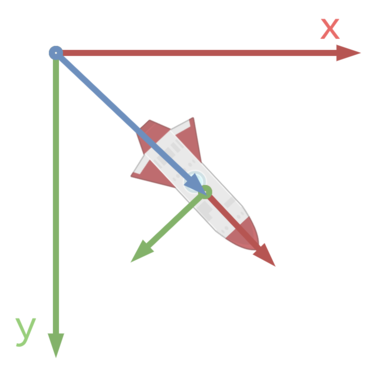### Converting Between Local and Global Space

You can convert coordinates from local to global by applying the transform. To apply a transform, use `xform()`:

``````    var global_position = transform.xform(local_position)
``````

For convenience, `Node2D` and `Spatial` include helper functions for this. Instead of using `transform.xform()`, you can call `to_global()`:

``````    var global_position = to_global(local_position)
``````

To convert from global to local coordinates, you can use the opposite (inverse) of the transform with `xform_inv()`.

Let’s use the example of an object in the 2D plane and convert mouse clicks (global space) into coordinates relative to the object:

``````extends Sprite

func _unhandled_input(event):
if event is InputEventMouseButton and event.pressed:
if event.button_index == BUTTON_LEFT:
printt(event.position, transform.xform_inv(event.position))
``````

As with the previous operation, there are helper functions for this. Instead of using `transform.xform_inv()`, you can call `to_local()`:

``````    printt(event.position, to_local(event.position))
``````

See the Transform2D docs for a list of the available properties and methods.

## 3D Transforms

In 3D space, the concept of transforms applies in the same way as in 2D. In fact, it becomes even more necessary, as using angles in 3D can lead to a variety of problems, as we’ll see in a bit.

3D nodes inherit from the base node Spatial, which contains the transform information. The 3D transform requires more information than the 2D version. Position is still held in the `origin` property, but rotation is in a property called `basis`, which contains three unit vectors representing the body’s local X, Y, and Z axes.As in 2D, we can use the local axes to move an object forward. In Godot’s 3D orientation (Y-up), this means that by default the body’s -Z axis is the forward direction:

``````    translation += -transform.basis.z
``````